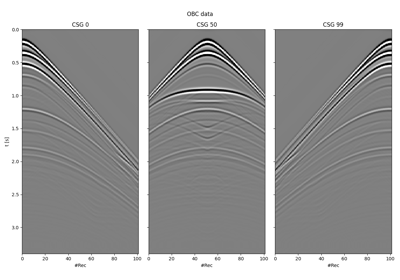# pylops.waveeqprocessing.BlendingGroup#

pylops.waveeqprocessing.BlendingGroup(nt, nr, ns, dt, times, group_size, n_groups, nproc=1, dtype='float64', name='B')[source]#

Group blending operator

Blend seismic shot gathers in group blending mode based on pre-defined sequence of firing times. In group blending a number of spatially closed sources are fired in a short interval. These sources belong to one group. The next group of sources is fired after all the data of the previous group has been recorded. The size of input model vector must be $$n_s \times n_r \times n_t$$, whilst the size of the data vector is $$n_{groups} \times n_r \times n_{t,tot}$$.

Parameters
ntint

Number of time samples

nrint

nsint

Number of sources. Equal to group_size x n_groups

dtfloat

Time sampling in seconds

timesnp.ndarray

Absolute ignition times for each source. This should have dimensions $$n_{groups} \times group_{size}$$, where each row contains the firing times for every group.

group_sizeint

The number of sources per group

n_groupsint

The number of groups

nprocint, optional

Number of processors used when applying operator

dtypestr, optional

Operator dtype

namestr, optional

Name of operator (to be used by pylops.utils.describe.describe)

Returns
Boppylops.LinearOperator

Blending operator

Notes

Simultaneous shooting or blending is the process of acquiring seismic data firing consecutive sources at short time intervals (shorter than the time requires for all significant waves to come back from the Earth interior).

Group blending refers to an acquisition scenario where two or more sources are towed behind a single vessel and fired at short time differences. The same experiment is repeated $$n_{groups}$$ times to create $$n_{groups}$$ blended recordings. For the case of 2 sources and an overall number of $$N=n_{groups}*group_{size}$$ shots, the modelling operator is

$\begin{split}\Phi = \begin{bmatrix} \Phi_1 & \Phi_2 & \mathbf{0} & \mathbf{0} & ... & \mathbf{0} & \mathbf{0} \\ \mathbf{0} & \mathbf{0} & \Phi_3 & \Phi_4 & ... & \mathbf{0} & \mathbf{0} \\ ... & ... & ... & ... & ... & ... & ... \\ \mathbf{0} & \mathbf{0} & \mathbf{0} & \mathbf{0} & ... & \Phi_{N-1} & \Phi_{N} \end{bmatrix}\end{split}$

where each $$\Phi_i$$ operator applies a time-shift equal to the absolute ignition time provided in the variable times.

## Examples using pylops.waveeqprocessing.BlendingGroup#Blending

Blending